Your data analysis solution

# Quantile regression in Excel tutorial

2018-07-20

This tutorial will help you set up and interpret a Quantile regression in Excel using the XLSTAT software.
Not sure this is the modeling feature you are looking for? Check out this guide

## Dataset for running a Quantile Regression

An Excel sheet with both the data and results used in this tutorial can be downloaded by clicking here.

The data have been obtained in Lewis T. and Taylor L.R. (1967). Introduction to Experimental Ecology, New York: Academic Press, Inc.. They concern 237 children, described by their Gender, Age in months, Height in inches (1 inch = 2.54 cm), and Weight in pounds (1 pound = 0.45 kg).

## Goal of this tutorial on Quantile Regression

Using the Quantile Regression, we want to find out how the weight of the children varies with their gender (a qualitative variable that takes value f or m), their height and their age, and to verify if a linear model makes sense. The Quantile Regression  method belongs to a larger family of models called GLM (Generalized Linear Models) as do the linear regression, the ANOVA and the ANCOVA.

The specificity of Quantile Regression with respect to these other methods is to provide an estimate of conditional quantiles of the dependent variable instead of conditional mean. In this way, Quantile Regression permits to give a more accurate quality assessment based on a quantile analysis.

The parameter estimates in QR linear models have the same interpretation as those of any other linear model, as rates of change. Therefore, in a similar way to the ordinary least squares (OLS) model, the coefficients of the QR model can be interpreted as the rate of change of some quantile of the dependent variable distribution per unit change in the value of some regressor.

Moreover, as in ANCOVA, it’s possible to mix qualitative and quantitative explanatory variables. In three other tutorials on linear regression this dataset is also used, with the Height (Linear Regression), the Height and the Age (ANOVA) and then the Height, the Age and the Gender (ANCOVA) as explanatory variables.

## Setting up a Quantile Regression

After opening XLSTAT, select the XLSTAT / Modeling data / Quantile Regression command (see below).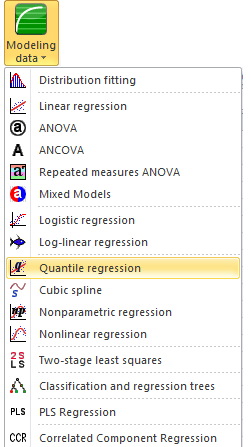Once you've clicked on the button, the Quantile Regression dialog box appears. Select the data on the Excel sheet. The Dependent variable (or variable to model) is here the Weight.

The quantitative explanatory variables are the height and the age. The qualitative variable is the gender. As we selected the column title for the variables, we leave the option Variable labels activated. The other options have been left at their default value.The computations begin once you have clicked on OK. The results will then be displayed.

In this study, we want to focus the analysis on quantiles for which the coefficients of the quantile regression are quite far from those of the ANCOVA.

In the following example, since no a-priori information giving quantiles of interest are assumed, a two-step analysis of the data is preferred and produced.

First, as an exploratory step, the Quantile Process can be selected to get an overview and thus detect some quantiles of interest to focus on next.

Before beginning, we recall the main results of ANCOVA applied on this dataset :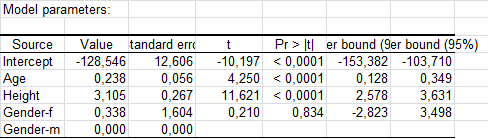## Interpreting the results of the first step of Quantile Regression : the Quantile Process computation

For this preliminary step, only general results are supplied (more options are available in the second step).

First, it can be noticed that around the median, the results are of the same order than those of the ANCOVA (for the mean) :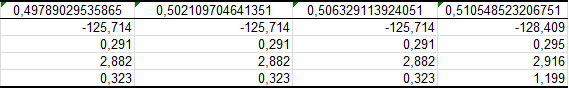Now, if we focus on the Age and Height, their contribution seems to be stronger in the evaluation of the weight of the biggest children (alpha>0.9):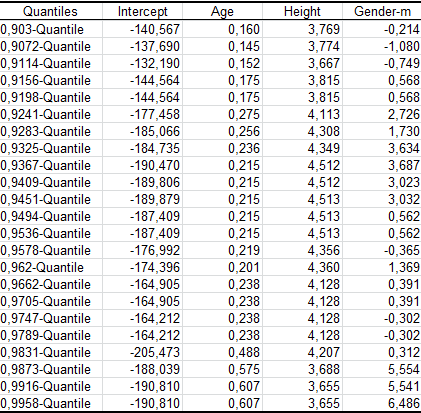whereas some other quantile regression results suggest that the Gender has a greater effect on the weight of the slimest ones (alpha<0.1):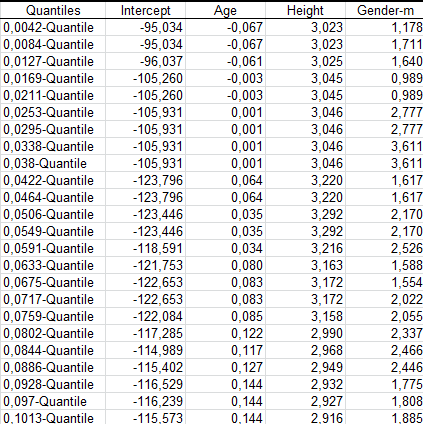Obviously,  the quantiles we are interested in belong to the intervals [0.9 , 1] and [0, 0.1].

It’s also easy and quick to confirm these impressions visualizing the illustrative charts displayed at the end of the analysis :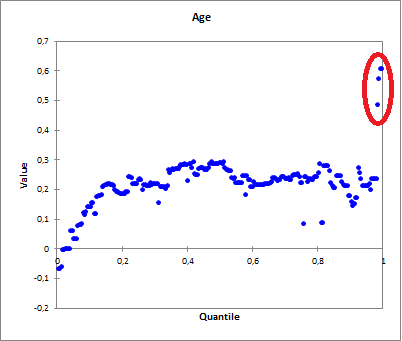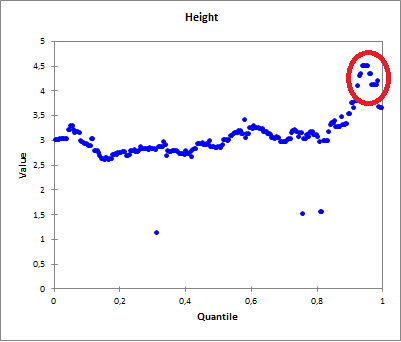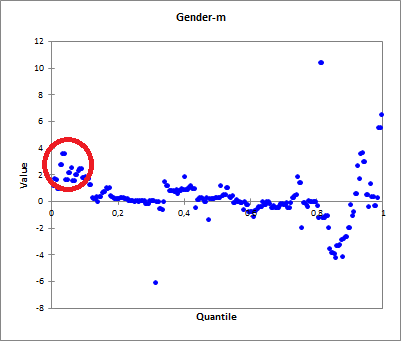Then, in a second step, the quantile Selection : 0.95 and 0.05 is done via the Excel sheet.

## Interpreting the results of the second step of Quantile Regression: the Quantile Selection computation

This first table displays the goodness of fit coefficients of the model for a specific quantile. The R² (coefficient of determination) indicates the % of variability of the dependant variable which is explained by the explanatory variables. The closer to 1 the R² is, the better the fit.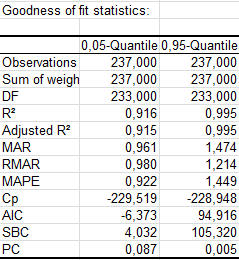In this particular case, 91.6 % of the variability of the Weight is explained by the Height, the Age and the Gender. The remainder of the variability is due to some effects (other explanatory variables) that have not been or that could not be measured during this experiment. We can guess that some genetic and nutritive effects are involved, but it might be that simply by transforming the available variables we could obtain some better results.

It is important to examine the results of the model significance table (see below). The results enable us to determine whether or not the explanatory variables bring significant information (null hypothesis H0) to the model. In other words, it's a way of asking yourself whether it is valid to use this quantile to describe the whole population, or whether the information brought by the explanatory variables is of value or not.Three tests are used : Maximum Likelihood, Lagrange Multipliers and Wald. Given the fact that the probability corresponding to the Chi² value is lower than 0.0001, it means that we would be taking a lower than 0.01% risk in assuming that the null hypothesis (no effect of the two explanatory variables) is wrong. Therefore, we can conclude with confidence that the three variables do bring a significant amount of information.

The following table gives details on the model. This table is helpful when predictions are needed, or when you need to compare the coefficients of the model for a given population with the ones obtained for another population.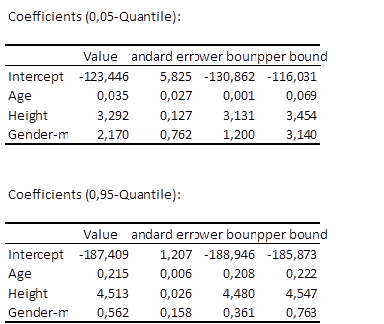The next table shows a part of the predictions and the residuals. It enables us to take a closer look at each of the standardized residuals.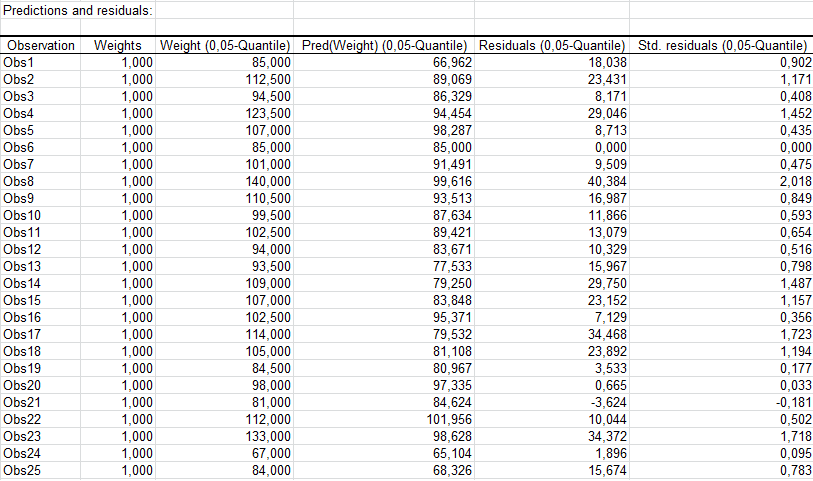The chart below shows the predicted values versus the observed values :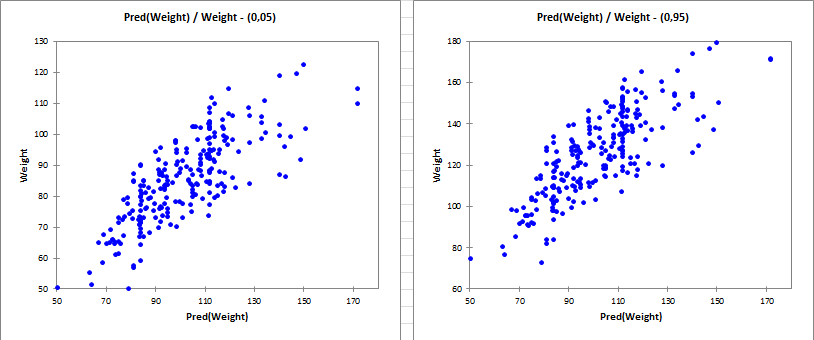## Conclusion for this Quantile Regression

As a conclusion, the Height, the Age and the Gender allow us to explain more than 90% of the variability of the Weight. A significant amount of information is explained by the Quantile Regression model we have used.

### Contact Us

#### Contact our technical support team: support@xlstat.com

https://cdn.desk.com/
false
desk
Loading
seconds ago
a minute ago
minutes ago
an hour ago
hours ago
a day ago
days ago
about
false
Invalid characters found
/customer/portal/articles/autocomplete
9283